Courses

# Test: Pure Substances - 2

## 30 Questions MCQ Test RRB JE for Mechanical Engineering | Test: Pure Substances - 2

Description
This mock test of Test: Pure Substances - 2 for Mechanical Engineering helps you for every Mechanical Engineering entrance exam. This contains 30 Multiple Choice Questions for Mechanical Engineering Test: Pure Substances - 2 (mcq) to study with solutions a complete question bank. The solved questions answers in this Test: Pure Substances - 2 quiz give you a good mix of easy questions and tough questions. Mechanical Engineering students definitely take this Test: Pure Substances - 2 exercise for a better result in the exam. You can find other Test: Pure Substances - 2 extra questions, long questions & short questions for Mechanical Engineering on EduRev as well by searching above.
QUESTION: 1

### In the figure shown, the system is a pure substance kept in a piston-cylinder arrangement. The system is initially a two-phase mixture containing 1 kg of liquid and 0.03 kg of vapour at a pressure of 100 kPa. Initially, the piston rests on a set of stops, as shown in the figure. A pressure of 200 kPa is required to exactly balance the weight of the piston and the outside atmospheric pressure. Heat transfer takes place into the system until its volume increases by 50%. Heat transfer to the system occurs in such a manner that the piston, when allowed to move, does so in a very slow (quasi-static I quasi-equilibrium) process. The thermal reservoir from which heat is transferred to the system has a temperature of 400°C. Average temperature of the system boundary can be taken as 17°C. The heat transfer to the system is I kJ, during which its entropy increases by 10 J/K. Atmospheric pressure.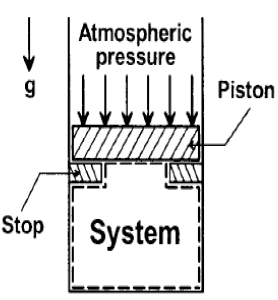Specific volumes of liquid (vf) and vapour (vg) phases, as well as values of saturation temperatures, are given in the table below.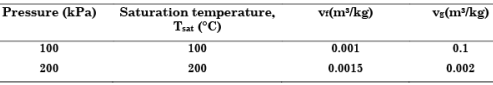The work done by the system during the process is:

Solution:

Work done = first constant volume heating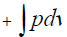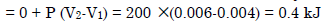QUESTION: 2

Solution:
QUESTION: 3

### The following table of properties was printed out for saturated liquid and saturated vapour of ammonia. The titles for only the first two columns are available. All that we know is that the other columns (columns 3 to 8) contain data on specific properties, namely, internal energy (kJ/kg), enthalpy (kJ/kg) and entropy (kJ/kgK)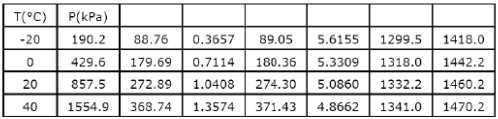When saturated liquid at 40°C is throttled to -20°C, the quality at exit will be

Solution: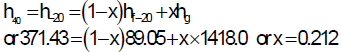QUESTION: 4

When an ideal gas with constant specific heats is throttled adiabatically, with negligible changes in kinetic and potential energies

Where h, T and S represent respectively, enthalpy, temperature and entropy, temperature and entropy

Solution: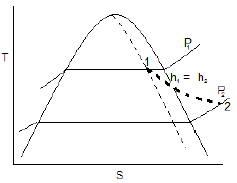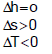QUESTION: 5

Assertion (A): Water is not a pure substance.

Reason (R): The term pure substance designates a substance which is homogeneous and has the same chemical composition in all phases.

Solution:

Water for all practical purpose can be considered as pure substance because it is homogeneous and has same chemical composition under all phases.

QUESTION: 6

The ordinate and abscissa in the given figure showing the saturated liquid and vapour regions of a pure substance represent: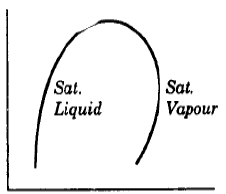Solution:

The ordinate and abscissa in given figure are pressure and enthalpy. Such diagram is common in vapour compression refrigeration systems.

QUESTION: 7

Consider the following statements for a throttling process:

1. It is an adiabatic process.

2. There is no work transfer in the process.

3. Entropy increases in throttling process

Which of these statements are correct ?

Solution:
QUESTION: 8

Consider the following:

1. Air

2. Gaseous combustion products

3. Steam

Which of these are pure substances, assuming there is no phase change?

Solution:

A pure substance is one whose chemical composition does not change during thermodynamic processes.

•  Pure Substance is one with uniform and invariant chemical composition.
•  Eg: Elements and chemical compounds are pure substances. (water, stainless steel)
•  Mixtures are not pure substances. (eg: Humid air)
•  Exception!! Air is treated as a pure substance though it is a mixture of gases.

Gaseous combustion products are a mixture of gases and not a pure substance.

QUESTION: 9

Considers the following properties of vapour:

1. Pressure

2. Temperature

3. Dryness fraction

4. Specific volume

Which of these two properties alone are not sufficient to specify the condition of a vapour?

Solution:
QUESTION: 10

Which p–v diagram for steam illustrates correctly the isothermal process undergone by wet steam till it becomes superheated?

Solution:

Up to saturation point pressure must be constant. After saturation its slope will be –ive, as pv = RT or pv = const. or vdp + pdv = 0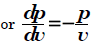QUESTION: 11

Consider the phase diagram of a certain substance as shown in the given figure. Match List-I (Process) with List-II (Curves/lines) and select the correct answer using the codes given below the lists: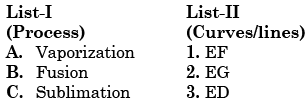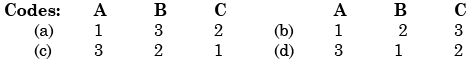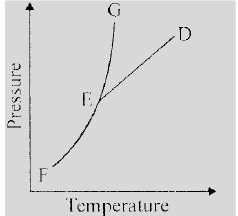Solution:
QUESTION: 12

The conversion of water from 40°C to steam at 200°C pressure of 1 bar is best represented as

Solution:
QUESTION: 13

The p-v-T surface of a pure substance is shown in the given p figure. The two-phase regions are labelled as: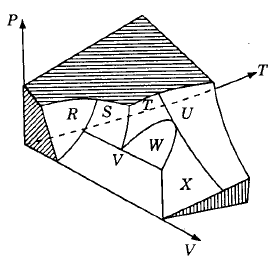Solution:
QUESTION: 14

Which one of the following is correct?

At critical point the enthalpy of vaporization is

Solution:

Characteristics of the critical point
1. It is the highest temperature at which the liquid and vapour phases can coexist.
2. At the critical point hfg, ufg and vfg are zero.
3. Liquid vapour meniscus will disappear.
4. Specific heat at constant pressure is infinite.

QUESTION: 15

Which one of the following statements is correct when saturation pressure of a vapour increases?

Solution:
QUESTION: 16

Which one of the following represents the condensation of a mixture of saturated liquid and saturated vapour on the enthalpy-entropy diagram?

Solution: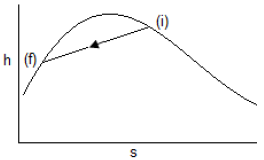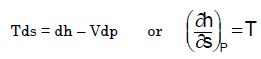The slope of the isobar on the h–s diagram is equal to the absolute temp, for condensation T is cost so slope is const, but not zero so it is inclined line.

QUESTION: 17

Consider the following statements regarding the throttling process of wet steam:

1. The steam pressure and temperature decrease but enthalpy remains constant.

2. The steam pressure decreases, the temperature increases but enthalpy remains constant.

3. The entropy, specific volume, and dryness fraction increase.

4. The entropy increases but the volume and dryness fraction decrease.

Which of the above statements are correct?

Solution:
QUESTION: 18

Consider the following statements:

When dry saturated steam is throttled from a higher pressure to a lower pressure, the

1. Pressure decreases and the volume increases

2. Temperature decreases and the steam becomes superheated

3. Temperature and the dryness fraction increase

4. Entropy increases without any change in enthalpy

Which of these statements are correct?

Solution:

Temperature decreases and the
steam becomes superheated.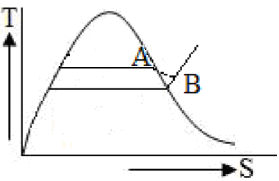QUESTION: 19

A fluid flowing along a pipe line undergoes a throttling process from 10 bar to 1 Bar in passing through a partially open valve. Before throttling, the specific volume of the fluid is 0.5 m3 /kg and after throttling is 2.0 m3 /kg. What is the Change in specific internal energy during the throttling process?

Solution:

Throttling is a isenthalpic process

h1 = h2 or u1 + p1v1 = u2 + p2v2 or u2 – u1 = p1v1 – p2v2 = 1000 × 0.5 – 100 × 2 = 300 kJ/kg

QUESTION: 20

In the figure shown, throttling process is represented by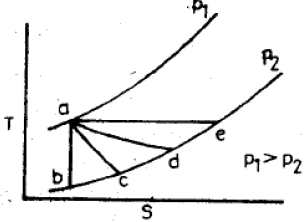Solution:
QUESTION: 21

Assertion (A): Air, a mixture of O2 and N2, is a pure substance.

Reason(R): Air is homogeneous in composition and uniform in chemical aggregation.

Solution:

A pure substance is a substance of constant chemical composition throughout its mass.

QUESTION: 22

Assertion (A): Air is a pure substance but a mixture of air and liquid air in a cylinder is not a pure substance.

.Reason (R): Air is homogeneous in composition but a mixture of air and liquid air is heterogeneous.

Solution:
QUESTION: 23

Two-phase regions in the given pressure-volume diagram of a pure substance are represented by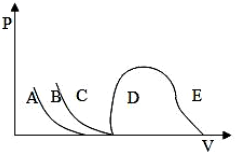Solution:
QUESTION: 24

Triple point temperature of water is:

Solution:

Remember: Triple point temperature of water = 273.16 K = 0.01°C

QUESTION: 25

Entropy of a saturated liquid at 227°C is 2.6 kJ/kgK. Its latent heat of vaporization is 1800 kJ/kg; then the entropy of saturated vapour at 227°C would be:

Solution: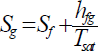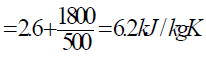QUESTION: 26

A piston –cylinder device contains 0.06m3 of saturated water vapour at 350 kPa pressure. Determine the temperature and mass of the vapour inside the cylinder.

Solution:

T = Tsat@350kPa = 138.86°C
v = vg@350kPa = 0.52422 m3/kg
m = V/v = 0.06 m3/0.52422 m3/kg = 0.114 kg.

QUESTION: 27

Constant pressure lines in the superheated region of the Mollier diagram have what type of slope?

Solution:

Mollier diagram is a h-s plot.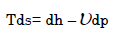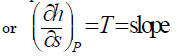T is always + ive so slope always +ive. Not only this if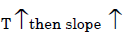QUESTION: 28

Dryness fraction of steam means the mass ratio of

Solution:
QUESTION: 29

In the following P-T diagram of water showing phase equilibrium lines, the sublimation line is: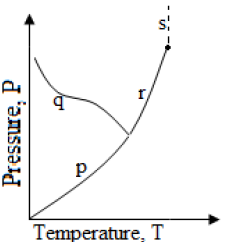Solution:

(a) p (b) q (c) r (d) S

QUESTION: 30

Assertion (A): In Mollier chart for steam, the constant pressure lines are straight lines in wet region.

Reason (R): The slope of constant pressure lines in wet region is equal to T.

Solution:

Track your progress, build streaks, highlight & save important lessons and more!

### Similar Content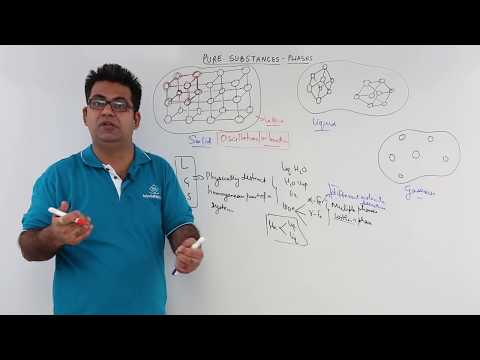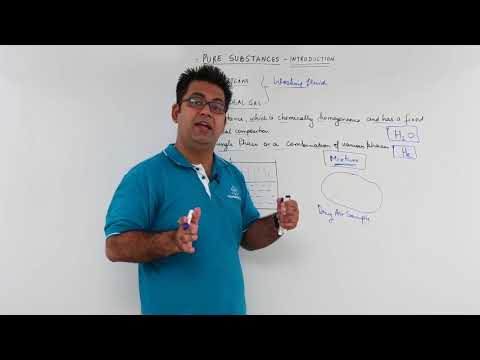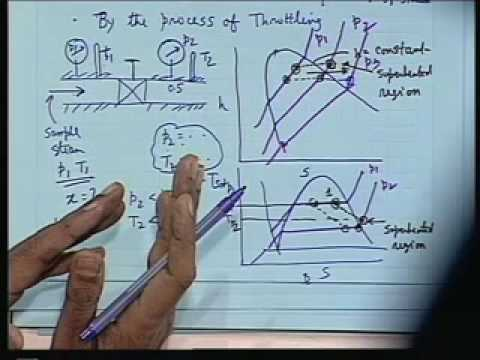### Related tests